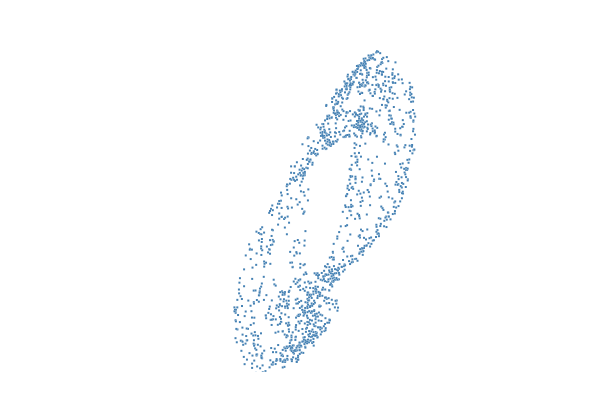# Cyclooctane

The conformation space of cyclooctane

Cyclooctane (CH₂)₈ is a molecule that consists of eight carbon atoms aligned in a ring, and eight hydrogen atoms, each of which is attached to one of the carbon atoms. The distance $c>0$ between neighboring carbon atoms is fixed.

In this example, we want to study the conformation space of cyclooctane with Homotopy Continuation. The equations for the positions $z_i\in\mathbb{R}^3$ of the carbon atoms satisfy the algebraic equations

$$\Vert z_1-z_2\Vert^2 = \cdots = \Vert z_7-z_8\Vert^2=\Vert z_8-z_1\Vert^2 = c^2.$$

The energy of a configuration $z=(z_1,\ldots,z_8)$ is minimized when the angles between successive bonds are all equal to $\text{arccos}(-\frac{1}{3}) \approx 109.5^\circ$. If we assume this geometrical constraint, by the law of cosines, the $z_i$ also satisfy the following equations:

$$\Vert z_1-z_3\Vert^2 = \cdots = \Vert z_6-z_8\Vert^2=\Vert z_7-z_1\Vert^2 =\Vert z_8-z_2\Vert^2= \frac{8c^2}{3}.$$

It is known that the solution set of these equations, up to simultaneous translation and rotation of the $z_i$, is homeomorphic to a union of the Klein bottle and a sphere, which intersect in two rings.

In this example, we demonstrate how to obtain points from the cyclooctane variety, which then can be further processed using, for instance, persistent homology and Ripser.

Let's define the equations in Julia for $c^2 = 2$. For this, we use the following normalization: since the equation of cyclooctane are invariant under simultaneous translation and rotation of the $z_i$, we define $z_1$ to be the origin, $z_8=(c,0,0)$ and $z_7$ to be rotated, such that its last entry is equal to zero. Thus we get a system of equations in $17$ variables.

using HomotopyContinuation, LinearAlgebra

c² = 2
@var z[1:3, 1:6]
z_vec = vec(z)[1:17] # the 17 variables in a vector
Z = [zeros(3) z[:,1:5] [z[1,6]; z[2,6]; 0] [√c²; 0; 0]] # the eight points in a matrix

# define the functions for cyclooctane:
F1 = [(Z[:, i] - Z[:, i+1]) ⋅ (Z[:, i] - Z[:, i+1]) - c² for i in 1:7]
F2 = [(Z[:, i] - Z[:, i+2]) ⋅ (Z[:, i] - Z[:, i+2]) - 8c²/3 for i in 1:6]
F3 = (Z[:, 7] - Z[:, 1]) ⋅ (Z[:, 7] - Z[:, 1]) - 8c²/3
F4 = (Z[:, 8] - Z[:, 2]) ⋅ (Z[:, 8] - Z[:, 2]) - 8c²/3
f = System([F1; F2; F3; F4])


The plan is now to intersect f=0 with a linear space of codimension $2$ many times to get points. The guide Solving many systems in a loop explains how to do this. We follow this guide and first generate a complex start system.

N = 17 # ambient dimension
L₀ = rand_subspace(N; codim = 2)
R_L₀ = solve(f; target_subspace = L₀)

Result with 1408 solutions
==========================
• 32768 paths tracked
• 1408 non-singular solutions (0 real)
• random_seed: 0x9edd6171
• start_system: :polyhedral


Now, we track the solutions from R_L₀ towards systems we are interested in (here is a quick comment for algebraic geometers: the size of R_p₀ is 1408; in other words, the cyclooctane variety has degree 1408).

# we compute 100 random intersections
Ω = solve(
f,
solutions(R_L₀);
start_subspace = L₀,
target_subspaces = [rand_subspace(N; codim = 2, real = true) for _ in 1:100],
transform_result = (R,p) -> real_solutions(R),
flatten = true
)


Now, Ω contains points from the cyclooctane variety. Here is a gif that shows 4470 points from the cyclooctane variety, projected onto a random three dimensional linear space.It is also possible to control the distribution of the points obtained by intersecting with linear spaces. We discuss this in this example.

Cite this example:
@Misc{ cyclooctane2022 ,
author =  { Paul Breiding },
title = { Cyclooctane },
howpublished = { \url{ https://www.JuliaHomotopyContinuation.org/examples/cyclooctane/ } },
note = { Accessed: June 27, 2022 }
}